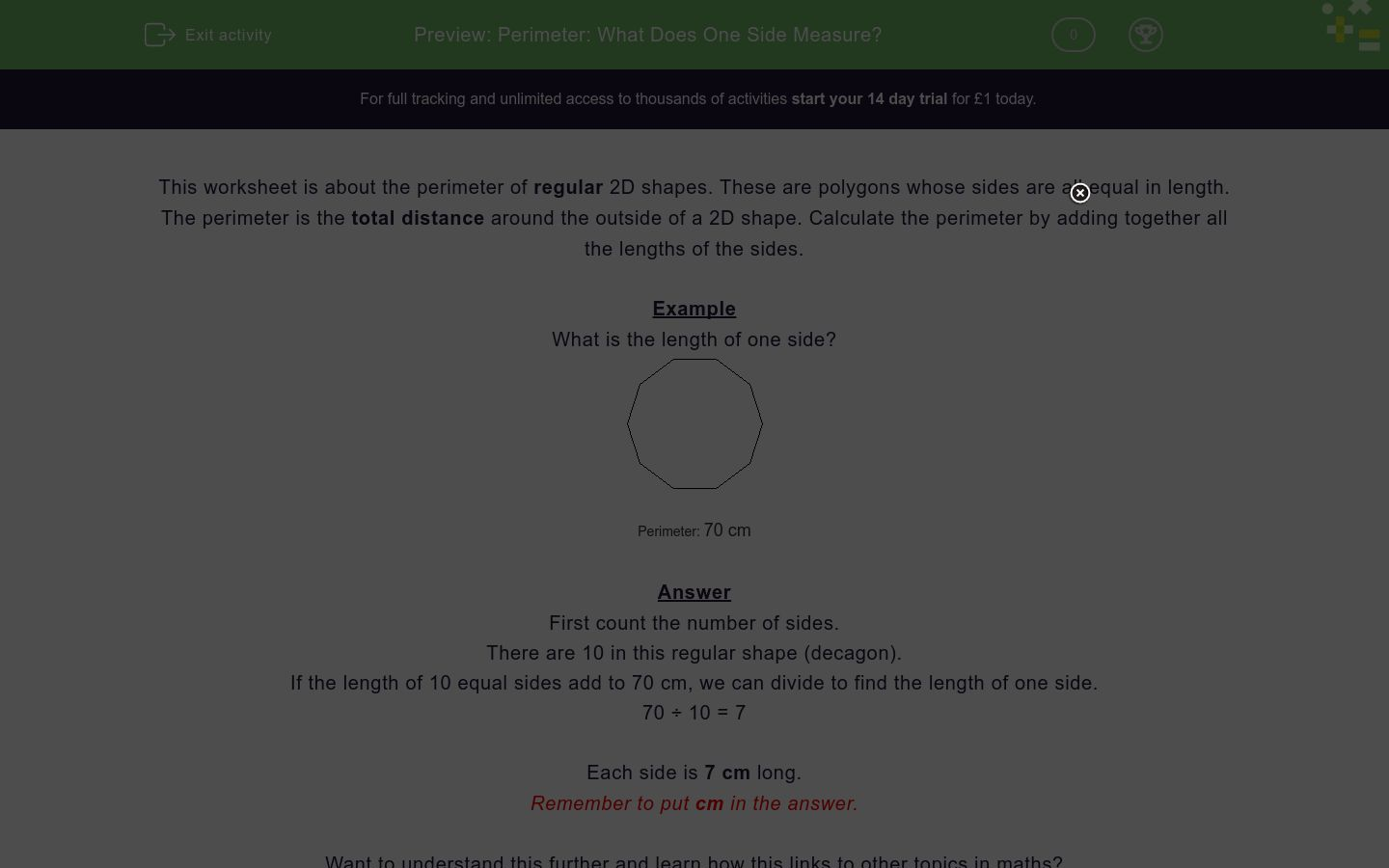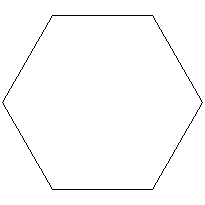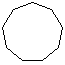# Perimeter: What Does One Side Measure?

In this worksheet, students calculate the length of one side of a regular polygon by first counting the number of sides and then dividing.Key stage:  KS 2

Curriculum topic:  Measurement

Curriculum subtopic:  Measure and Calculate Shapes

Difficulty level:### QUESTION 1 of 10

This worksheet is about the perimeter of regular 2D shapes.  These are polygons whose sides are all equal in length.

The perimeter is the total distance around the outside of a 2D shape. You calculate it by adding together all the lengths of the sides.

Example

What is the length of one side?Perimeter: 70 cm

First count the number of sides.

There are 10 in this regular shape (decagon)

If the length of 10 equal sides add to 70 cm, we can divide to find the length of one side.

70 ÷ 10 = 7

Each side is 7 cm long.

What is the length of one side?Perimeter: 20 cm

What is the length of one side?Perimeter: 60 cm

What is the length of one side?Perimeter: 80 cm

What is the length of one side?Perimeter: 27 cm

What is the length of one side?Perimeter: 48 cm

What is the length of one side?Perimeter: 90 cm

What is the length of one side?Perimeter: 60 cm

What is the length of one side?Perimeter: 63 cm

What is the length of one side?Perimeter: 72 cm
• Question 1

What is the length of one side?Perimeter: 20 cm
4cm
4 cm
• Question 2

What is the length of one side?Perimeter: 60 cm
10 cm
10cm
• Question 3

What is the length of one side?Perimeter: 80 cm
10cm
10 cm
• Question 4

What is the length of one side?Perimeter: 27 cm
3cm
3 cm
• Question 5

What is the length of one side?Perimeter: 48 cm
6 cm
6cm
• Question 6

What is the length of one side?Perimeter: 90 cm
10cm
10 cm
• Question 7

What is the length of one side?Perimeter: 60 cm
6 cm
6cm
• Question 8

What is the length of one side?Perimeter: 63 cm
9 cm
9cm
• Question 9

What is the length of one side?Perimeter: 72 cm